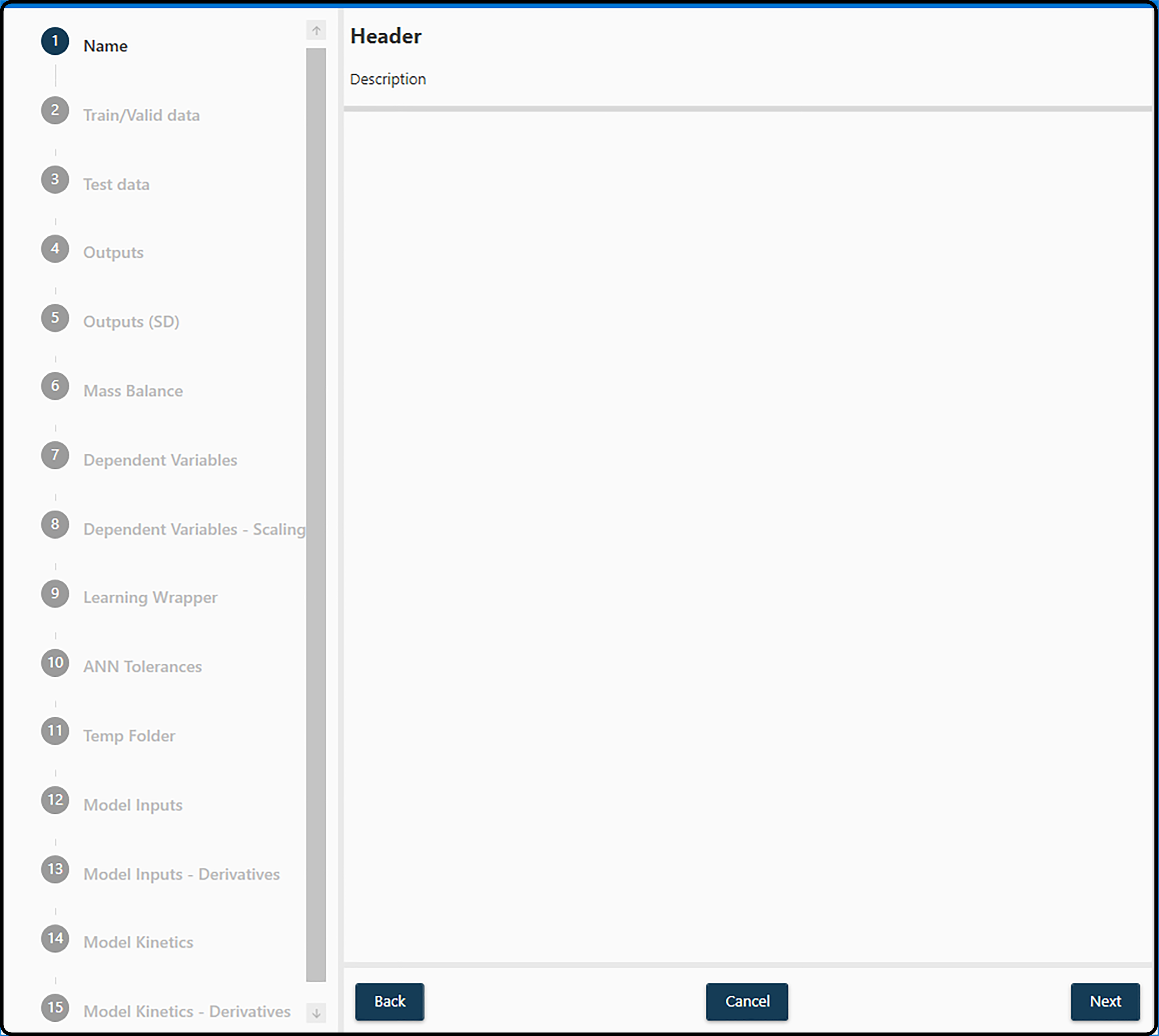# Identification¶

## Overview - creating a hybrid model¶

After creating a new project and adding data to it, a hybrid model can be set up via PROJECT and Add Hybrid Model. This opens a new dialog, in which several parameters have to be specified:Figure 18. Setting up a hybrid model starting with a model name and ending with the specification of the model kinetics.

Here only a very short description is given for each required item, details can be found in the respective subchapters.

1. Name: a model name (see section Model Name).

2. Train/Valid Data: the data set(s) to be used for setting up and optimizing the hybrid model.

3. Test Data: the data set, on which the final model shall be applied to estimate its preformance.

4. Outputs: one or more variables in the data set serving as response, i.e. the quantity to predict.

5. Outputs (SD):

6. Mass Balance: to properly setup a hybrid model, quantities like feed, sampling volume or reactor volume are required and need to be specified at this point.

7. Dependent Variables: inputs to the artificial neural network (ANN) model

8. Dependent Variables – scaling:

9. Learning Wrapper: (technical) model details, such as the ANN model complexity (number of hidden layers, number of hidden nodes, …) or the validation method

10. ANN Tolerances:

11. Temp Folder:

12. Model Inputs: specify the variables in the data set to enter the neural network model as inputs. Also simple mathematical functions of those can be used, such as $$ln(x_1 + x_2)$$ or similar.

13. Model Inputs – Derivatives:

14. Model Kinetics: the kinetic expressions in the form of the right hand side of the differential equations for the state variables (model outputs).

15. Model Kinetics – Derivatives:

Completing these steps creates a hybrid model and adds it to the current selected project. Selecting it in the corresponding section on the left and pressing Start will start the optimization process.

Note

Several parameters requested in the dialog above can still be changed/modified at this step.

Note

If the model creation failed, check the console log for possible errors.